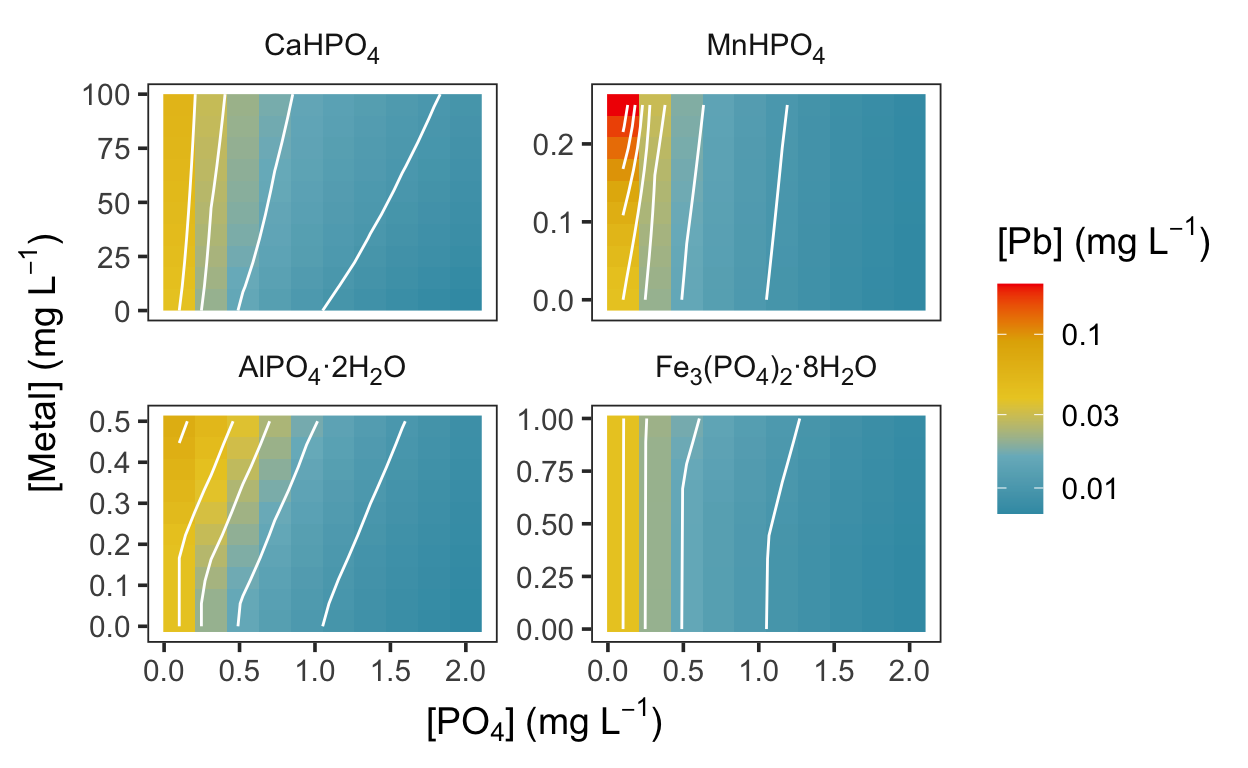# New preprint!

Aluminum in drinking water can interact with orthophosphate, increasing lead solubility.

Ben Trueman true
08-13-2021

Recently, my coauthors and I used a preprint server (chemRxiv) to share an early draft of a research paper for the first time. Preprints are not very common in my field, but I imagine that will change in the future as their advantages become clear. The paper, “Seasonal lead release to drinking water and the effect of aluminum” (Trueman et al. 2021), explores the role of aluminum in limiting the availability of orthophosphate for lead corrosion control in drinking water systems.

Orthophosphate works by forming an insoluble precipitate with lead, but precipitation with other metals can limit its effect on lead solubility. Here, I reproduce some of the code included in the paper to account for aluminum phosphate precipitation in calculating equilibrium lead solubility. Solubility modeling is done here using `pbcusol`, an interface for PHREEQC in R that is geared specifically to lead and copper solubility predictions. I also extend the analysis to include other metals that might precipitate with orthophosphate and interfere with lead phosphate formation.

The concentrations of interfering aluminum, calcium, manganese, and iron have been chosen to approximate typical ranges, although more extreme values are certainly possible or even likely. A caveat: the phases that precipitate in the model and limit available orthophosphate—variscite (AlPO4·2H2O), vivianite (Fe3(PO4)2·8H2O, CaHPO4, and MnHPO4—may not be the phases that precipitate in drinking water systems.

To reproduce the model, load the necessary packages and define a few chemical equations that are not included in the default (`pbcusol`) database.

``````library("tidyverse")
# remotes::install_github("bentrueman/pbcusol)
library("pbcusol")

solids <- list(
"Variscite",
"AlPO4:2H2O = Al+3 + PO4-3 + 2H2O",
"log_k" = -22.36, # https://doi.org/10.1016/j.gca.2010.10.012
"CaHPO4",
"CaHPO4 = Ca+2 + H+ + PO4-3",
"log_k" = -19.275 # from phreeqc::minteq.v4.dat
)

aqueous_species <- list(
# from https://doi.org/10.1016/j.gca.2010.10.012 and
# https://doi.org/10.1080/09593332708618735
"HPO4-2 + Al+3 = AlHPO4+",
"log_k" = 7.4,
"HPO4-2 + H+ + Al+3 = AlH2PO4+2",
"log_k" = 3.1
)
``````

Then, define a couple of functions that we will use to build the model. The first is a wrapper around `pbcusol::eq_sol_fixed()` that saves having to code the same arguments repeatedly. The second builds a list of the arguments to `pbcusol::eq_sol_fixed()` that change across iterations.

``````pb_sol_custom <- function(interference = "Variscite", ...) {
pbcusol::eq_sol_fixed(element = "Pb",
ph = 7.3, dic = 5,
Na = 10 / chemr::mass("Na"),
eq_phase_components = rlang::list2(!!interference := c(0, 0)),
new_species = aqueous_species,
new_phase = solids,
...
) %>%
select(pb_ppb)
}

build_args <- function(x) {
list(x\$metal_conc / chemr::mass(x\$metal)) %>%
set_names(nm = x\$metal) %>%
c(list(
phase = x\$phase,
interference = x\$interference,
phosphate = x\$po4
))
}
``````

Lead solubility is calculated over a grid of input values in parallel, using `future::plan()`.

``````grid_size <- 10

future::plan("multisession") # parallel iteration

out <- crossing(
phase = c("Hxypyromorphite", "Cerussite"),
po4 = seq(0.1, 2, length.out = grid_size),
metal = c("Ca", "Al", "Mn", "Fe"),
metal_conc = seq(0, .5, length.out = grid_size)
) %>%
mutate(
interference = fct_recode(metal,
"Variscite" = "Al", "MnHPO4(C)" = "Mn",
"Vivianite" = "Fe", "CaHPO4" = "Ca"
) %>%
as.character(),
# adjust metal concentrations to approximate typical values:
metal_conc = case_when(
metal == "Ca" ~ metal_conc * 200,
metal == "Fe" ~ metal_conc * 2,
metal == "Mn" ~ metal_conc * .5,
TRUE ~ metal_conc
)
) %>%
rowid_to_column() %>%
group_by(rowid) %>%
nest() %>%
ungroup() %>%
mutate(
args = map(data, build_args),
pb_ppb = furrr::future_map(args, ~ do.call(pb_sol_custom, .x))
)
``````

And here are the results. At pH 7.3 and a dissolved inorganic carbon concentration of 5 mg C L-1, all four metals impact lead solubility to some extent, and the predicted effect of manganese is largest.Trueman, Benjamin F, Aaron Bleasdale-Pollowy, Javier A Locsin, Jessica L Bennett, Wendy H Krkošek, and Graham A Gagnon. 2021. “Seasonal Lead Release to Drinking Water and the Effect of Aluminum.” Preprint. Chemistry. https://doi.org/10.33774/chemrxiv-2021-59nd6-v2.

### Corrections

If you see mistakes or want to suggest changes, please create an issue on the source repository.

### Citation

`Trueman (2021, Aug. 13). Benjamin Trueman: New preprint!. Retrieved from https://bentrueman.github.io/posts/2021-08-13-new-preprint/`
```@misc{trueman2021new,